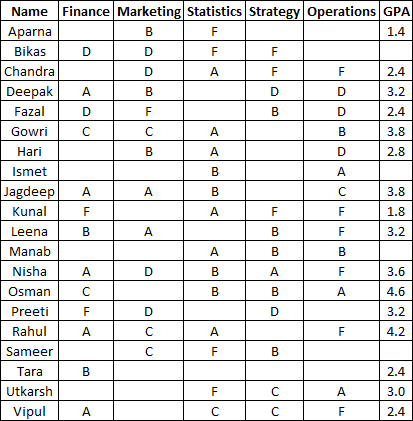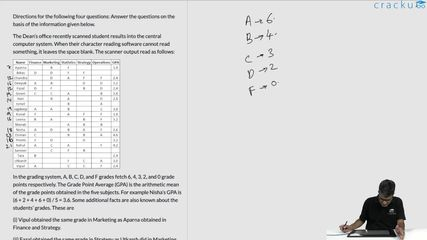### CAT 2004 Question Paper Question 36

Instructions

Directions for the following four questions: Answer the questions on the basis of the information given below.

The Dean’s office recently scanned student results into the central computer system. When their character reading software cannot read something, it leaves the space blank. The scanner output read as follows:In the grading system, A, B, C, D, and F grades fetch 6, 4, 3, 2, and 0 grade points respectively. The Grade Point Average (GPA) is the arithmetic mean of the grade points obtained in the five subjects. For example Nisha’s GPA is (6 + 2 + 4 + 6 + 0) / 5 = 3.6. Some additional facts are also known about the students’ grades. These are

(i) Vipul obtained the same grade in Marketing as Aparna obtained in Finance and Strategy.

(ii) Fazal obtained the same grade in Strategy as Utkarsh did in Marketing.

Question 36

# What grade did Preeti obtain in Statistics?

Solution

Let preeti obtain x and y in stastistics and operation respectively. Now we know that GPA = 3.2 .

So (x+y+2+2) = 16 .

So x+y = 12. for this only one value is possible x=y=6 .

### View Video Solution